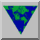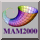# Math Makes Points

Locating points precisely on a map or in the ocean or within a microscopic sample requires careful measurements. Once we have a collection of such data, we can try to find patterns that indicate relationships. When the points are on a line or stretched out along a curve, we only need one number to locate each point. Once we choose a single point we can identify other points just by saying how far they are from this point along the curve. Points on a map require two numbers, for example latitude and longitude provided by some Global Positioning System. Points above or below the surface of the earth require three coordinates, not just latitude and longitude by height (measured by positive numbers) or depth, measured by negative numbers.

Any time we make a survey or take a sample, we collect data points. Some of these might have more than one or two numbers for each observation, maybe more than three or four. Mathematicians still want to look for patterns, and here the computer can be a tremendous help. We can show two or three variables at a time to obtain a swarm of points on a computer screen. Sometimes as we move these around, patters will appear that were not evident in the original tables of data points.

The subject that investigates data in this way is called Exploratory Data Analysis. One of the pioneers in this important field is John Tukey, an applied mathematician who did much of his work at Princeton University and AT&T Bell Laboratories.

A point has only one property, its location. It is a mathematical abstraction, with no length, width, or height, no thickness or volume. We say that a point has zero dimensions, even though it might take one or more numbers to specify the location of the point. For example, the origin of a number line is a particularly important point, identified by the number 0. Other mathematically important points are the center of a circle or the corners of a square.

A zero-dimensional object is a collection of points, for example the two endpoints of a line segment, or the three vertices of a triangle. The only measure associated with a finite set of points is the number of points. Recently there have been entire books devoted to the number zero, for example The Nothing that Is; A Natural History of Zero by Robert Kaplan and Zero: The Biography of a Dangerous Idea by Charles Seife.

From a geometric viewpoint, that number specifies the origin of a number line, but from the point of view of arithmetic, it serves as a place holder in our decimal notation, in a number like 2000 for example. The fact that we can write a number like 1999 as 1000 + 900 + 90 + 9 is crucial in our understanding of the algorithm for multiplication, as explained in the recent thesis of Li Ping Ma, Knowing and Teaching Elementary Mathematics: Teachers' Understanding of Fundamental Mathematics in China and the United States.

The long history of the number 0 is a fascinating study in the way mathematics is developed, and the importance of numeration systems in furthering out understanding of science. A point in the plane can be specified by two numbers, the coordinates with respect to any pair of lines intersecting at their mutual origin. In three-space, we need three coordinates for every point, and so on into spaces of four dimensions and higher. So the location of a point can be specified by any number of coordinates, although the point itself has zero dimension.Math Awareness Month 2000 Last modified: Mar 23, 2001 3:43:18 PM Comments to: `thomas_banchoff@brown.edu` Hosted by: The Math Forum